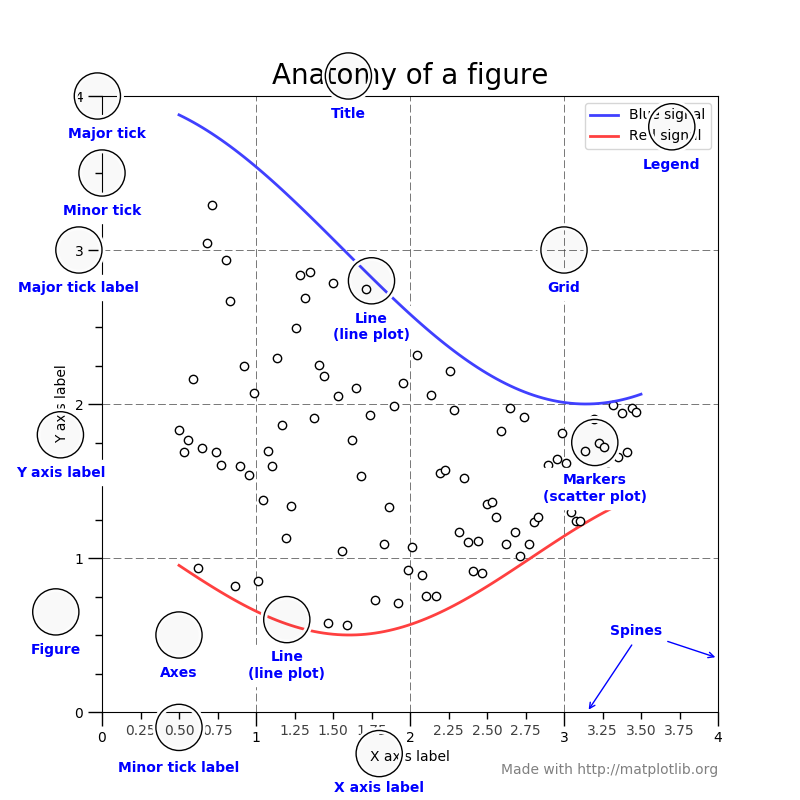# Matplotlib

## A way to draw things in python which is better than no way to draw things in python.

The classic python plotting is matplotlib. It can’t do all those modern hipster graphs without hard labour and is awful at animations and interactions, and it fugly per default. It works OK out of the box. There are libraries which use matplotlib as a backend and build more eleaborate systems on the top, but these have not had much longevity so far, so I find myself falling back to plain old matplotlib. It is an acceptable default with lots of weird edge cases when you try to be clever, but gets the job 80% done.

Note some confusing terminology; An Axes object, which is constructed by an add_subplot command, contains two Axis objects, but is much more than a list of such objects, being the fundamental object upon which a graph is drawn.

But don’t listen to me describe it. Observe this lovely diagram which explains all..

Taxonomy of a matplotlib plot. I’ve lost the source for this image, sorry.

Here are some miscellaneous tips:

## Grammar of graphics

Plotnine implements a best-effort clone of R’s ggplot2 library for matplotlib I believe plotnine supersedes the abandoned(?) ggplot.py by yhat (ggplot source, plotnine source).

## Matplotlib styling

The default matplotlib stylesheet aspires to look like 80s spreadsheet defaults, but if you are not a retrofuturist, you want to change the stylesheet. Some of the built-in stylesheets are OK.

Seaborn is another vaunted extension, which I would describe as an “Edward Tufterizer”. Extends matplotlib with modern appearance and some missing plot types.

A cute hack to justify matplotlib’s existence: xkcd graphs.

### Giant margins on saved graphics

plt.savefig("image.png", dpi=300, bbox_inches='tight', pad_inches=0)

### Axis labels

Suppressing?

ax = plt.gca()
ax.axes.xaxis.set_visible(False)
ax.axes.yaxis.set_visible(False)

## image montage

I have an array of images in arr. How can I plot them on a nice simple plot? I need to do this all the time. If I have skimage installed I can use the montage function. I do not always have that installed though. Here is a snippet to do it by hand:

columns = 5
rows = 3
fsize = 6
fig = plt.figure(figsize=(fsize *columns/rows, fsize))

for i in range(1, columns*rows +1):
img = arr[1,:,:,i]
plt.imshow(img)
ax.set_axis_off()
plt.show()

## Fonts

Someone made the idiosyncratic choice that default font is sans serif, even for mathematical text. You can change this by setting serif fonts also for mathtext.

from matplotlib import rc
rc(
'font',
family='serif',
serif=['Palatino']
)
rc(
'mathtext',
fontset='cm'
)

Supported math fonts are reputedly

• dejavusans (horrible default)
• dejavuserif (beware of odd greek letters)
• cm (”Computer Modern”. Classic, dated. )
• stix
• stixsans (sounds like sans serif to me)

Alternatively you can render your graph labels with TeX which leads to some weird spacing but allows you to match fonts better. It is also fragile and character set issues are terrible. Is this better if I use XeLaTeX/LuaLaTeX?

I am indebted to my colleague Christian Wlder for suggesting this as a reliable initialisation procedure for matplotlib plotting.

mport matplotlib
import sys, os

# GTK GTKAgg GTKCairo MacOSX Qt4Agg TkAgg WX WXAgg CocoaAgg
# GTK3Cairo GTK3Agg WebAgg agg cairo emf gdk pdf pgf ps svg template

is_mac = sys.platform == 'darwin'
if is_mac:
_matplotlib_backend = 'MacOSX'
else:
_matplotlib_backend = 'pdf'

matplotlib.rcParams['svg.fonttype'] = 'none'
matplotlib.rcParams['backend'] = _matplotlib_backend
matplotlib.rcParams['mathtext.fontset'] = 'stix'
matplotlib.rcParams['font.family'] = 'Times New Roman'

matplotlib.use(_matplotlib_backend)
import matplotlib.pyplot as plt
import matplotlib as mpl

plt.switch_backend(_matplotlib_backend)
# print(matplotlib.pyplot.get_backend())
try:
import cairocffi as cairo
except:
pass
# logging.warning('import cairocffi failed')

_latex_preamble = [
r'\usepackage{amsmath,bm}',
r'\newcommand\what{\hat{\bm{w}}}',
r'\newcommand\tr{^\top}',
r'\newcommand\dt{\left|#1\right|}',]

_latex_path = '/Library/TeX/texbin/'

def use_latex_mpl(latex_path=_latex_path, latex_preamble=_latex_preamble):
mpl.rcParams['text.usetex'] = True
mpl.rcParams['text.latex.preamble'] = latex_preamble
if latex_path is not None:
os.environ['PATH'] = '%s:%s' % (os.environ['PATH'], latex_path)

## Yellowbrick

Yellowbrick is a matplotlib specialisation for hyperparameter optimisation.

Yellowbrick extends the Scikit-Learn API to make model selection and hyperparameter tuning easier. Under the hood, it’s using Matplotlib.

### No comments yet. Why not leave one?

GitHub-flavored Markdown & a sane subset of HTML is supported.